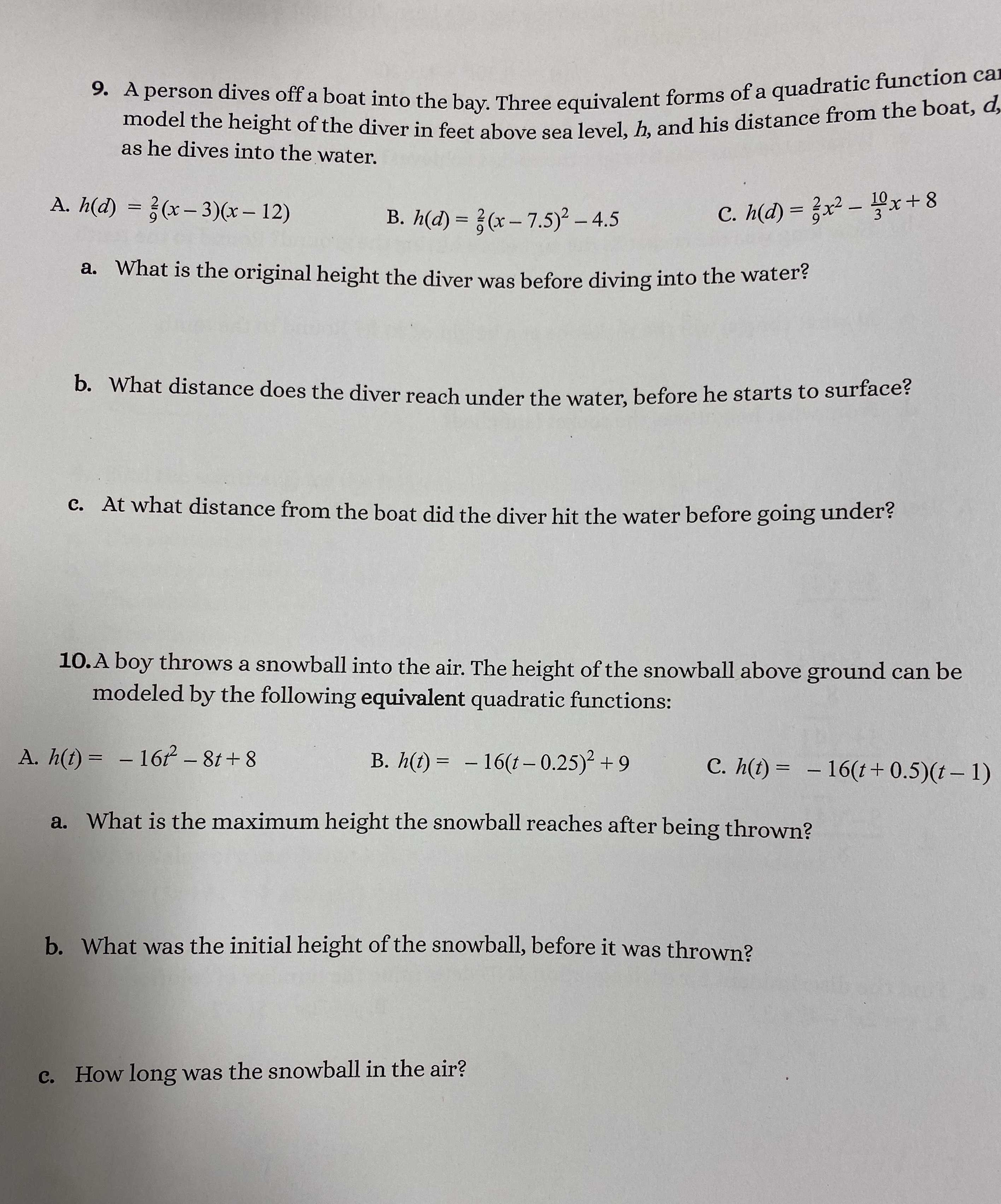### ¿Todavía tienes preguntas de matemáticas?

Pregunte a nuestros tutores expertos
Algebra
Pregunta9. A person dives off a boat into the bay. Three equivalent forms of a quadratic function car model the height of the diver in feet above sea level, $$h$$ , and his distance from the boat, $$d$$ , as he dives into the water.

A. $$h ( d ) = \frac { 2 } { 9 } ( x - 3 ) ( x - 12 )$$

B. $$h ( d ) = \frac { 2 } { 9 } ( x - 7.5 ) ^ { 2 } - 4.5$$

C. $$h ( d ) = \frac { 2 } { 9 } x ^ { 2 } - \frac { 10 } { 3 } x + 8$$

a. What is the original height the diver was before diving into the water?

b. What distance does the diver reach under the water, before he starts to surface?

c. At what distance from the boat did the diver hit the water before going under?

10. A boy throws a snowball into the air. The height of the snowball above ground can be modeled by the following equivalent quadratic functions:

A. $$h ( t ) = - 16 t ^ { 2 } - 8 t + 8$$

B. $$h ( t ) = - 16 ( t - 0.25 ) ^ { 2 } + 9$$

C. $$h ( t ) = - 16 ( t + 0.5 ) ( t - 1 )$$

a. What is the maximum height the snowball reaches after being thrown?

b. What was the initial height of the snowball, before it was thrown?

c. How long was the snowball in the air?

9.

a. 8 feet

b. 4.5 feet

c. 3 feet

10.

a. 9 feet

b. 8 feet

c. 1 second

Solución
View full explanation on CameraMath App.